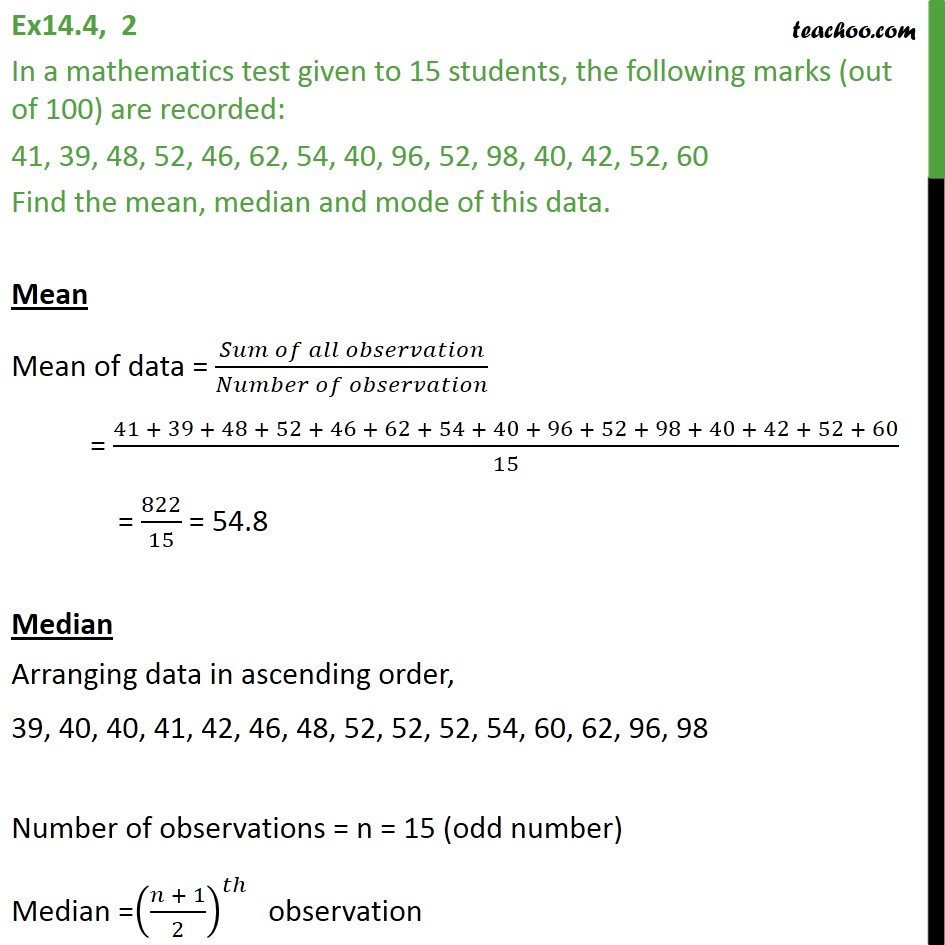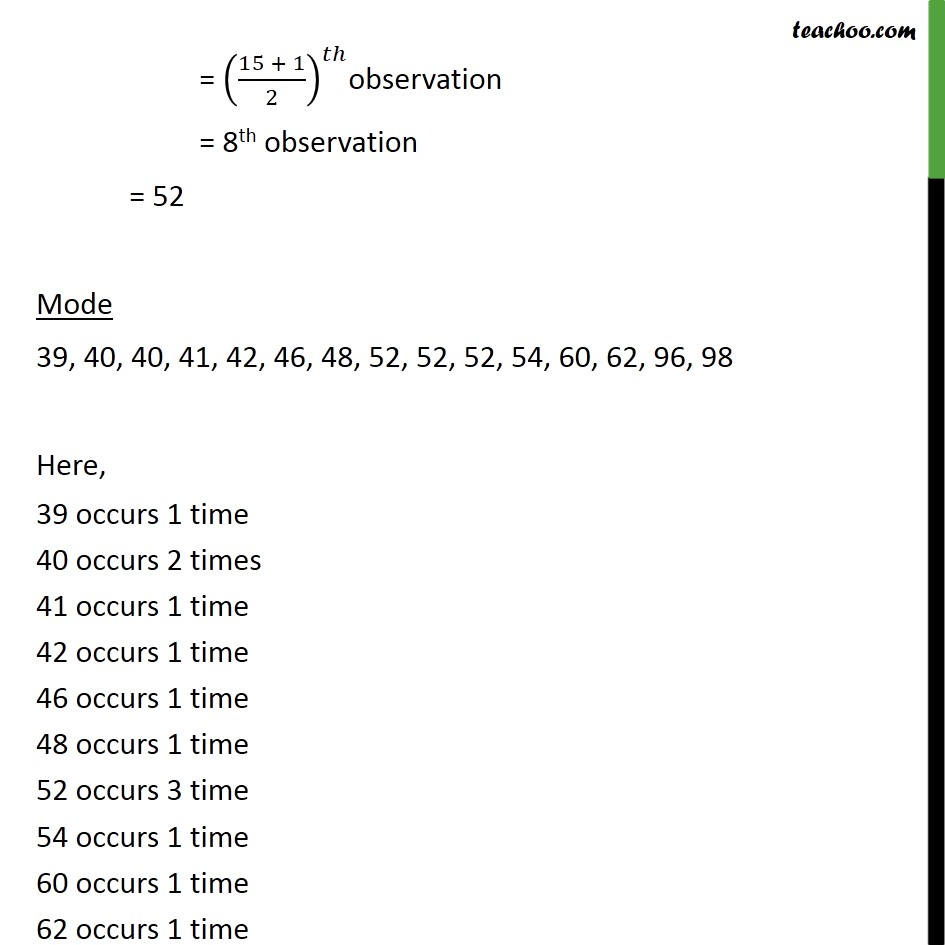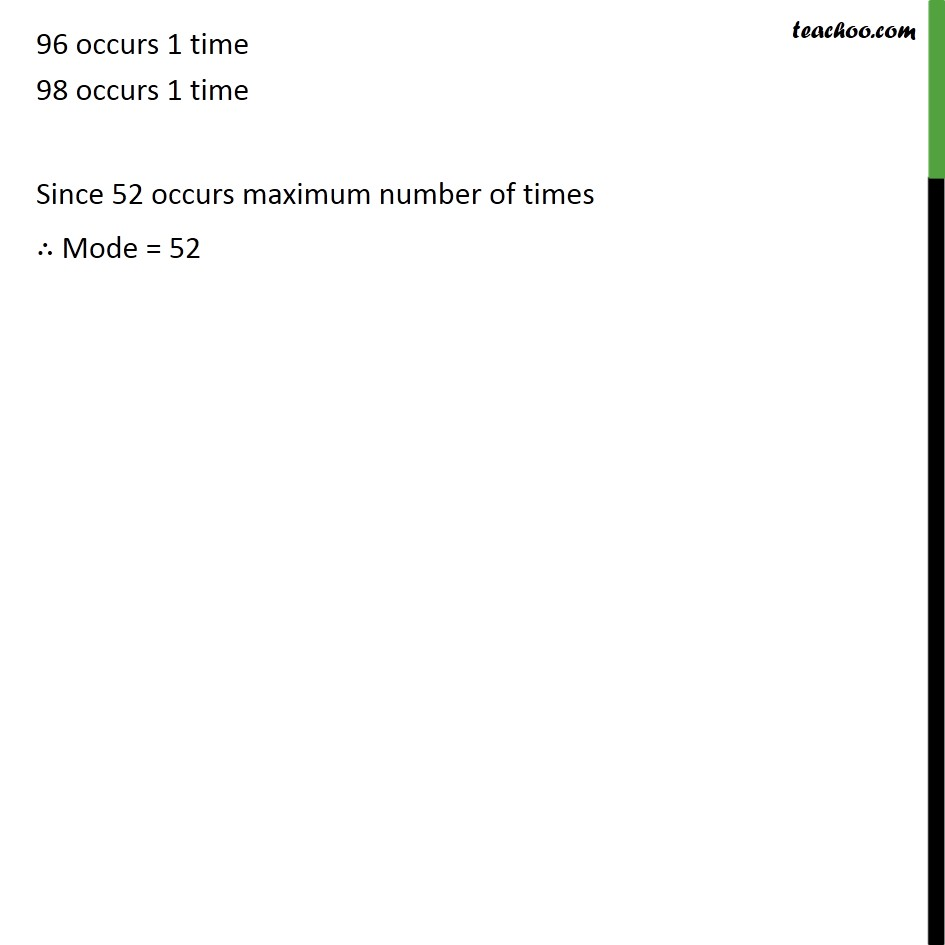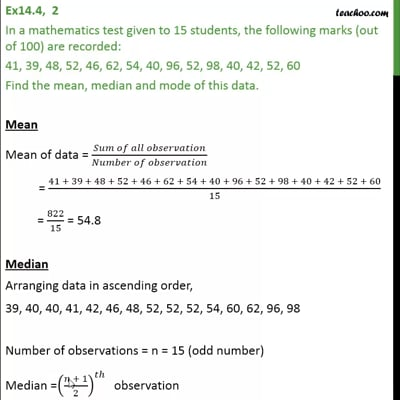Ex 14.4

Chapter 14 Class 9 Statistics (Term 1)
Serial order wiseThis video is only available for Teachoo black users

### Transcript

Ex14.4, 2 In a mathematics test given to 15 students, the following marks (out of 100) are recorded: 41, 39, 48, 52, 46, 62, 54, 40, 96, 52, 98, 40, 42, 52, 60 Find the mean, median and mode of this data. Mean Mean of data = ( )/( ) = (41 + 39 + 48 + 52 + 46 + 62 + 54 + 40 + 96 + 52 + 98 + 40 + 42 + 52 + 60)/15 = 822/15 = 54.8 Median Arranging data in ascending order, 39, 40, 40, 41, 42, 46, 48, 52, 52, 52, 54, 60, 62, 96, 98 Number of observations = n = 15 (odd number) Median =(( + 1)/2)^ " obs"ervation = ((15 + 1)/2)^ observation = 8th observation = 52 Mode 39, 40, 40, 41, 42, 46, 48, 52, 52, 52, 54, 60, 62, 96, 98 Here, 39 occurs 1 time 40 occurs 2 times 41 occurs 1 time 42 occurs 1 time 46 occurs 1 time 48 occurs 1 time 52 occurs 3 time 54 occurs 1 time 60 occurs 1 time 62 occurs 1 time 96 occurs 1 time 98 occurs 1 time Since 52 occurs maximum number of times Mode = 52